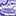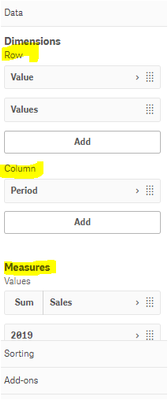App Development

Announcements
QlikWorld 2022, LIVE in Denver CO., May 16-19, 2022. REGISTER NOW TO RECEIVE EARLY BIRD PRICING
cancel
Showing results for
Did you mean:Creator

How to find out Average Earnings revenue in Last 7 days using SET Analysis in Qlik Sense?

Hi All,

I am facing an issue to find out estimated revenue in my sale with an average value i.e.

From below expression how to get an average of my revenue in %age form:

(
(
(
SUM({<Date = {"<=\$(vLast6Days))>=\$(vLast7Days)"}>}[Estimated Earnings])
-
SUM({<Date = {"<=\$(vLast5Days))>=\$(vLast6Days)"}>}[Estimated Earnings])
)
-
(SUM({<Date = {"<=\$(vCDplusOne)>=\$(vCurrentDay)"}>}[Estimated Earnings]))
)
/
(
SUM({<Date = {"<=\$(vLast6Days))>=\$(vLast7Days)"}>}[Estimated Earnings])
-
SUM({<Date = {"<=\$(vLast5Days))>=\$(vLast6Days)"}>}[Estimated Earnings])
)
)

*100

Labels (1)
• qlik sense set analysis

3 RepliesSpecialist

Hi,

1. Average of Estimated earnings=(Sum( {\$<Date = {“>=\$(=Today()-6)<=\$(Today())”} >} [Estimated Earnings] )/6)  Result from Example:5764.667

2. Average of Estimated earnings %=((Sum( {\$<Date = {“>=\$(=Today()-6)<=\$(Today())”} >} [Estimated Earnings] )/6) / (Sum( {\$<Date = {“>=\$(=Today()-6)<=\$(Today())”} >} [Estimated Earnings] )) * 100

Result from Ex:16.66667%

Ex:

 01-06-2020 02-06-2020 03-06-2020 04-06-2020 05-06-2020 06-06-2020 Sumup Sumup/6 Avg % 500 5667 8766 8978 9889 788 34588 5764.667 16.66667Creator
Author

How can I map date this.....in QS data load editor .Specialist

hi,

hi,

i have done in Pivot table,

you can use Dimension Column see below:Tags
Community Browser